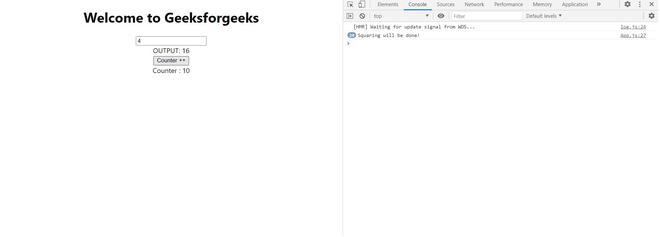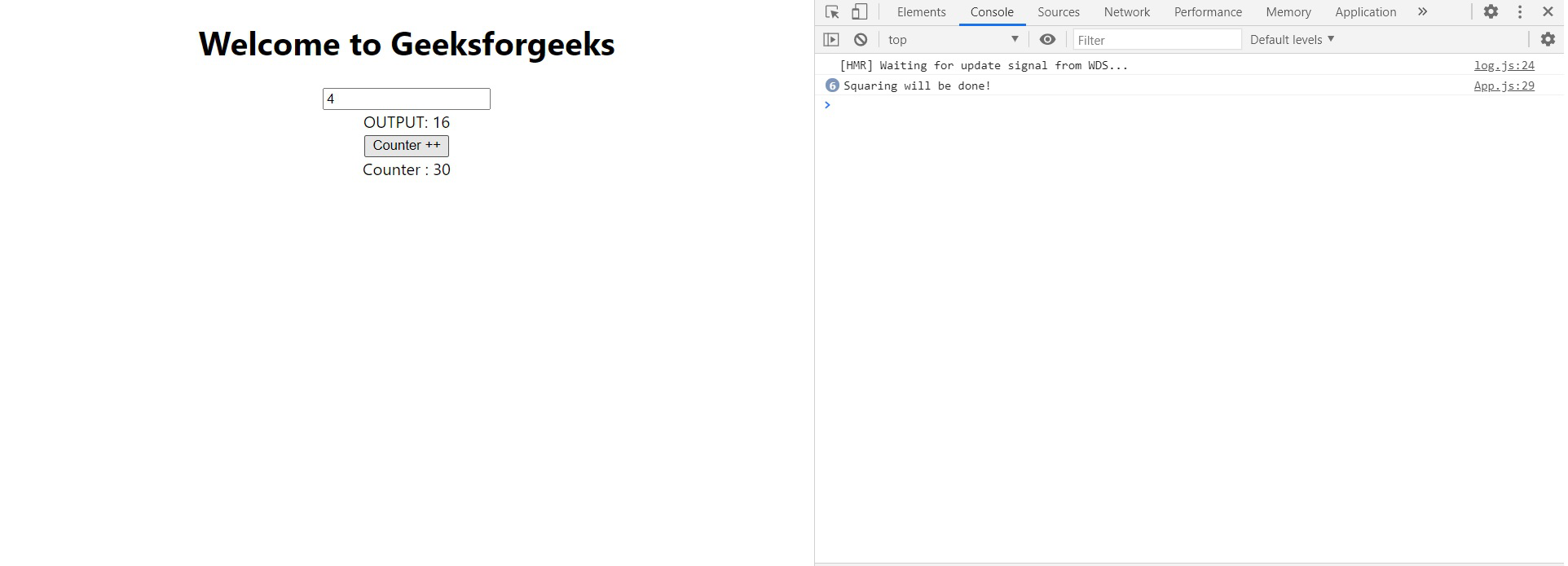# React JS useMemo Hook

• Difficulty Level : Medium
• Last Updated : 27 Oct, 2020

The useMemo is a hook used in the functional component of react that returns a memoized value. In Computer Science, memoization is a concept used in general when we don’t need to recompute the function with a given argument for the next time as it returns the cached result. A memoized function remembers the results of output for a given set of inputs. For example, if there is a function to add two numbers, and we give the parameter as 1 and 2 for the first time the function will add these two numbers and return 3, but if the same inputs come again then we will return the cached value i.e 3 and not compute with the add function again. In react also, we use this concept, whenever in the React component, the state and props do not change the component and the component does not re-render, it shows the same output. The useMemo hook is used to improve performance in our React application.

Syntax:

```const memoizedValue = useMemo(functionThatReturnsValue,
arrayDepencies)
```

Example: When we don’t use the useMemo Hook.

## Javascript

 `import React, {useState} from ``'react'``;`` ` `function` `App() {``  ``const [number, setNumber] = useState(0)``  ``const squaredNum =  squareNum(number);``  ``const [counter, setCounter] = useState(0);`` ` ` ``// Change the state to the input``  ``const onChangeHandler = (e) => {``    ``setNumber(e.target.value);``  ``}``   ` `  ``// Increases the counter by 1``  ``const counterHander = () => {``    ``setCounter(counter + 1);``  ``}``  ``return` `(``    ``
``      ``

Welcome to Geeksforgeeks

``      ````      ````       ` `      ``
OUTPUT: {squaredNum}
``      ````      ``
Counter : {counter}
``    ``
``  ``);``}`` ` `// function to square the value``function` `squareNum(number){``  ``console.log(``"Squaring will be done!"``);``  ``return` `Math.pow(number, 2);``}`` ` `export ``default` `App;`

Output: In the above example, we have an App component and this component is doing two things one is squaring a number on the given input and incrementing the counter. We have two states here number and counter, whenever any of the states change the component re-renders. For example, if we change the input value of the number the function squareNum runs, and if increment the counter again the function squareNum runs. We can see this on the console.In this case, we can see that even if we changed the input number once, but clicked on-increment counter multiple times our function squareNum got executed whenever we clicked the increment counter button multiple times. This is happening because the App component re-renders whenever we change the state of the counter.

Now let’s solve this problem using the useMemo hook.

Example: When we use useMemo Hook

## Javascript

 `import React, {useState} from ``'react'``;`` ` `function` `App() {``  ``const [number, setNumber] = useState(0)``  ``// Using useMemo``  ``const squaredNum = useMemo(()=> {``    ``return` `squareNum(number);``  ``}, [number])``  ``const [counter, setCounter] = useState(0);`` ` ` ``// Change the state to the input``  ``const onChangeHandler = (e) => {``    ``setNumber(e.target.value);``  ``}``   ` `  ``// Increases the counter by 1``  ``const counterHander = () => {``    ``setCounter(counter + 1);``  ``}``  ``return` `(``    ``
``      ``

Welcome to Geeksforgeeks

``      ````      ````       ` `      ``
OUTPUT: {squaredNum}
``      ````      ``
Counter : {counter}
``    ``
``  ``);``}`` ` `// function to square the value``function` `squareNum(number){``  ``console.log(``"Squaring will be done!"``);``  ``return` `Math.pow(number, 2);``}`` ` `export ``default` `App;`

Output: Now in the above example, we have used the user memo hook, here the function that returns the value i.e squareNum is passed inside the useMemo and inside the array dependencies, we have used the number as the squareNum will run only when the number changes. If we increase the counter and the number remains the same in the input field the squareNum doesn’t run again. Let’s see the output below.Now we can see in the console that the squareNum is running only when there is a change in the input box and not when the button of the increment counter is clicked.

Note: Memorization is never free, we are trading space for time.

My Personal Notes arrow_drop_up